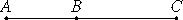# Proposition 40

If two straight lines incommensurable in square which make the sum of the squares on them medial but the rectangle contained by them rational are added together, then the whole straight line is irrational; let it be called the side of a rational plus a medial area.
X.34

Let two straight lines AB and BC incommensurable in square, and fulfilling the given conditions, be added together.

I say that AC is irrational.X.16

Since the sum of the squares on AB and BC is medial, while twice the rectangle AB by BC is rational, therefore the sum of the squares on AB and BC is incommensurable with twice the rectangle AB by BC, so that the square on AC is also incommensurable with twice the rectangle AB by BC.

X.Def.I.4

But twice the rectangle AB by BC is rational, therefore the square on AC is irrational. Therefore AC is irrational. Let it be called the side of a rational plus a medial area.

Q.E.D.

## Guide

This proposition is used in X.46, X.58, X.64, and X.69.